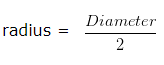# How to find Circumference of a Circle

Circumference of a circle is the measure of the length of the circle.

Circumference of a circle = 2πr

### How to find circumference of a circle when radius is given

Question 1

Find the circumference of a circle of radius 7 cm?

Explanation

The radius of given circle is r = 7 cm

Circumference of the circle = 2πr

= ( 2 x 22/7 x 7 ) cm

= 44 cm

Question 2

Find the circumference of a circle of radius 28 cm?

Explanation

The radius of given circle is r = 28 cm

Circumference of the circle = 2πr

= ( 2 x 22/7 x 28 ) cm

= 176 cm

Question 3

Find the circumference of a circle of radius 49 cm?

Explanation

The radius of given circle is r = 49 cm

Circumference of the circle = 2πr

= ( 2 x 22/7 x 49 ) cm

= 308 cm

Question 4

Find the circumference of a circle of radius 3.5 cm?

Explanation

The radius of given circle is r = 3.5 cm

Circumference of the circle = 2πr

= ( 2 x 22/7 x 3.5 ) cm

= 22 cm

How to find circumference of a circle when diameter is given

Question 1

Find the circumference of a circle, whose diameter is 14 cm?

Explanation

The diameter of given circle = 14 cm

Then,= 14/2 cm = 7 cm

Circumference of circle = 2πr

= ( 2 x 22/7 x 7 ) cm

= 44 cm

Question 2

Find the circumference of a circle, whose diameter is 56 cm?

Explanation

The diameter of given circle = 56 cm

Then,= 56/2 cm = 28 cm

Circumference of circle = 2πr

= ( 2 x 22/7 x 28 ) cm

= 176 cm

Question 3

Find the circumference of a circle, whose diameter is 140 cm?

Explanation

The diameter of given circle = 140 cm

Then,= 140/2 cm = 70 cm

Circumference of circle = 2πr

= ( 2 x 22/7 x 70 ) cm

= 440 cm

Question 4

Find the circumference of a circle, whose diameter is 98 cm?

Explanation

The diameter of given circle = 98 cm

Then,= 98/2 cm = 49 cm

Circumference of circle = 2πr

= ( 2 x 22/7 x 49 ) cm

= 308 cm